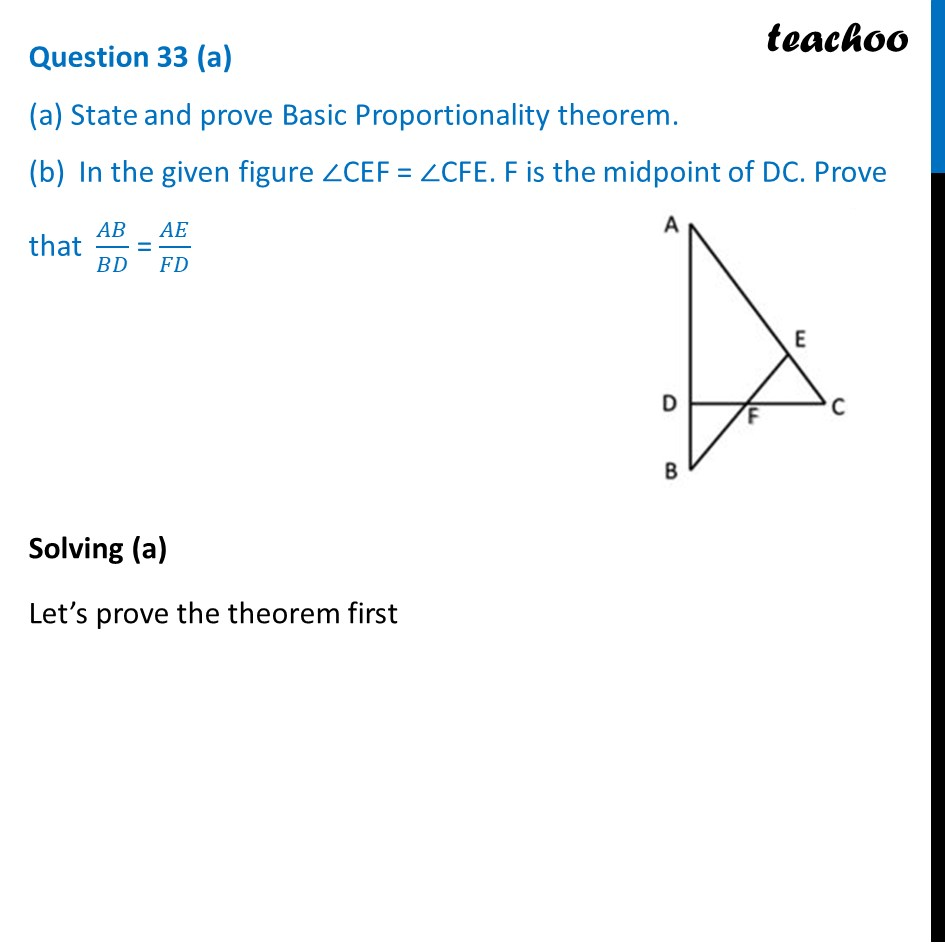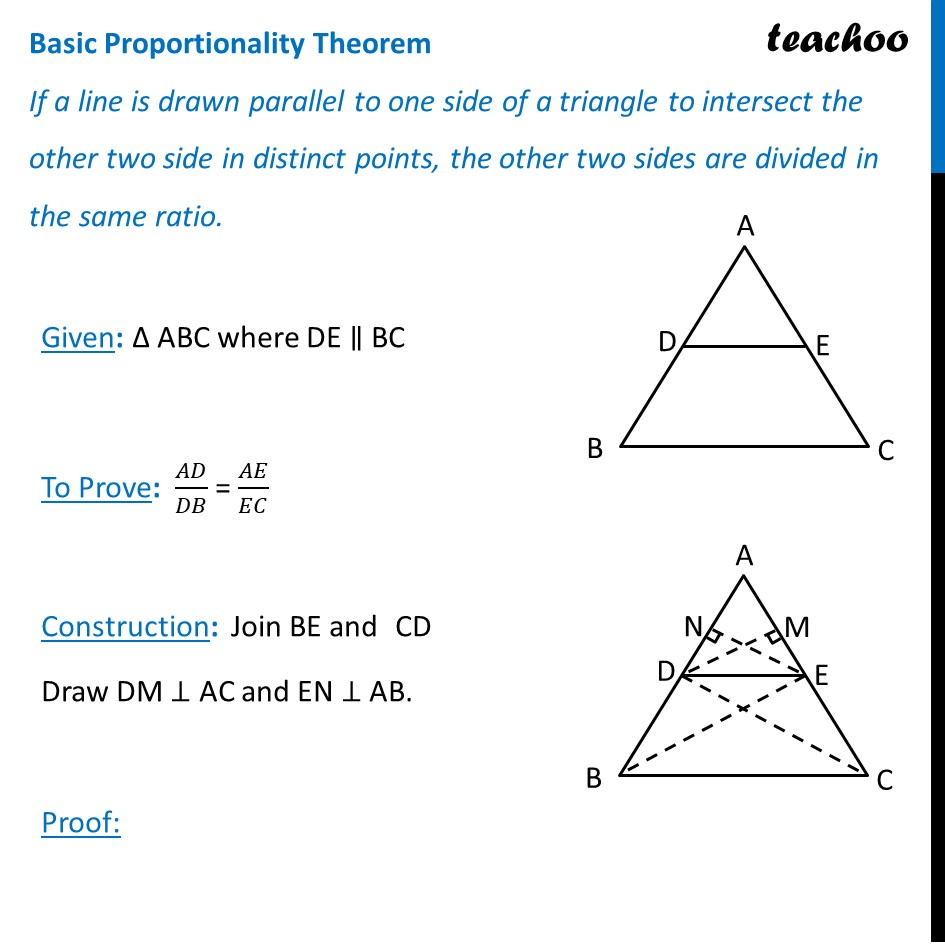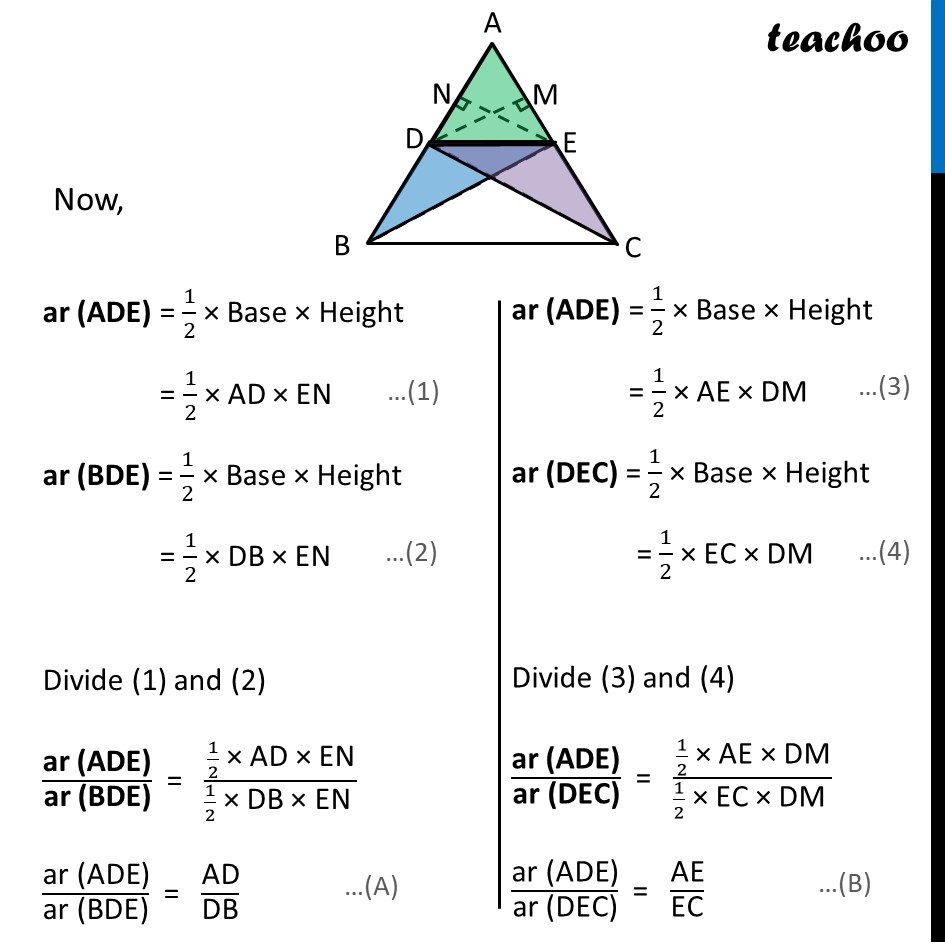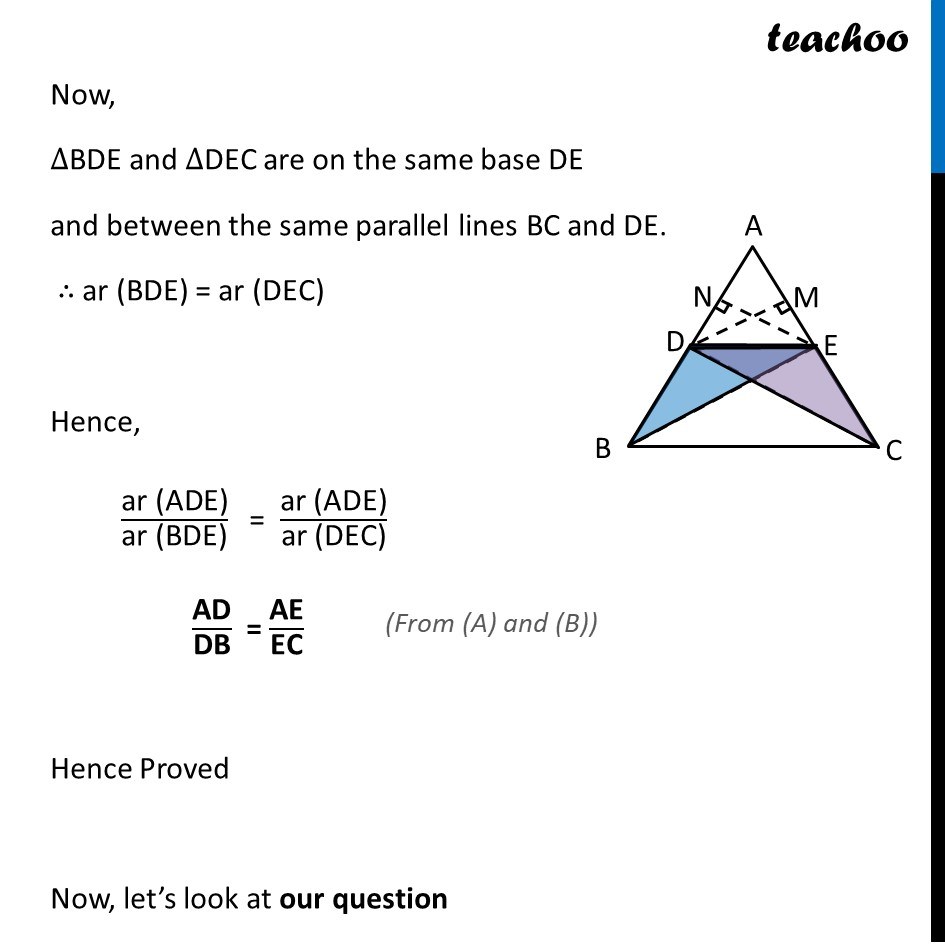CBSE Class 10 Sample Paper for 2024 Boards - Maths Standard

Class 10
Solutions of Sample Papers for Class 10 Boards

## (b)  In the given figure ∠CEF = ∠CFE. F is the midpoint of DC. Prove that  AB/BD = AE/FDLearn in your speed, with individual attention - Teachoo Maths 1-on-1 Class

### Transcript

Given: Δ ABC where DE ∥ BC To Prove: 𝐴𝐷/𝐷𝐵 = 𝐴𝐸/𝐸𝐶 Construction: Join BE and CD Draw DM ⊥ AC and EN ⊥ AB. Proof: ar (ADE) = 1/2 × Base × Height = 1/2 × AD × EN ar (BDE) = 1/2 × Base × Height = 1/2 × DB × EN Divide (1) and (2) "ar (ADE)" /"ar (BDE)" = (1/2 " × AD × EN" )/(1/2 " × DB × EN " ) "ar (ADE)" /"ar (BDE)" = "AD" /"DB" Now, ∆BDE and ∆DEC are on the same base DE and between the same parallel lines BC and DE. ∴ ar (BDE) = ar (DEC) Hence, "ar (ADE)" /"ar (BDE)" = "ar (ADE)" /"ar (DEC)" "AD" /"DB" = "AE" /"EC" Hence Proved Now, let’s look at our question